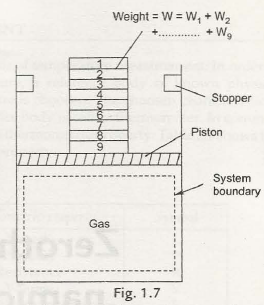The properties can only be defined when the states of the system possess a state of thermodynamic equilibrium. So it is evident that until the state of equilibrium occurs in the system, the intermediate states along the paths cannot take place. Sorting out an example might help you to understand the entire thing. Let us take a piston-cylinder mechanism as shown in figure 1.7 given below. Now the W weight is divided into several small weights like W1, W2, ……Wq. Now if the weights are removed one by one very slowly then the gas in the cylinder undergoes several changes of state. Thus all the states that occurinbetweenget deviated from equilibrium infinitesimal. This typical process is called quasistatic process or quasi-equilibrium process.Now if the total weight W is suddenly detached then the piston will automatically get a bump and will attack the stoppers. This condition will continue until it attains equilibrium even after many oscillations. However, this is not possible in this experiment that describes the intermediate states or paths. This is thus what called a non-equilibriumprocess.

Thus this is thequasi-staticprocess where thereis only an insignificant alteration in properties of matter from its equilibrium.

In several cases, one property remains constant during a process. The prefix iso is used to describe such a process. If thetemperature remains constant, the process is called isothermal process. If pressure remains constant, the process is known as isobaric or isopiestic process, and if volume is constant, it is called isochoric process.

Links of Next Mechanical Engineering Topics:-### Customer Reviews

My Homework Help
Rated 5.0 out of 5 based on 510 customer reviews at